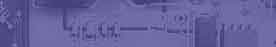Ohms law. Using Circuit Magic to teach Ohms law. Ohms symbol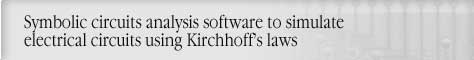Circuit analysis Tutorial
AKNM Circuit Magic- circuit analysis software

Ohm’s law

Ohm's law is the main basic electrical law and defines the resistance of a device to the flow of electrons.

There are three different notations of Ohm’s law

1.Unknown current

2.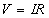Unknown voltage

3.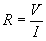Unknown resistance

(Most people can remember a picture easier than a mathematical formula. By knowing any two values you can figure out the third. Simply put your finger over the portion of the symbol you are trying to figure out and you have your formula)

1.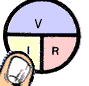2.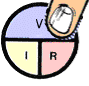3.